# Dash Adventures in a Fractional World

by  Wonder Workshop

## 1.1Description

Operations with fractions can be a tough concept for many students to grasp. In this lesson, students will learn how to multiply a whole number by a fraction using a number line and Dash for a visual representation. Students will then write word problems involving this concept, swap with a neighbor, and present the solution to the class using Dash as a visual representation.

Subjects: ELA, Math, Coding

·         Group Size: 1 - 4 students per Dash robot

·         Time Required: 2+ hours (4 lessons)

·         Online Lesson: Dash Adventures in a Fractional World

## 1.2        Objectives

·         Recognize that a fraction a/b is a multiple of 1/b.

·         Recognize that a multiple of a/b is also a multiple of 1/b, and use this understanding to multiply a fraction by a whole number.

·         Solve word problems involving multiplication of a fraction by a whole number, using visual fraction models and equations to represent the problem.

### 1.2.1Adaptations for Different Grades or Ability Levels

·         Students lower than grade 4 may have difficulty with this lesson. You may choose to only do activity 1 and 4, using very simple fractions such as 1/4, 2/4, 3/4.

·         Create more complex fractions and equations for older students, and less complex fractions and equations for younger students.  Match level to those covered in math textbooks.

·         Instead of you creating the programs, choose a few students who are comfortable with Blockly code to create the programs for the number line activities.  Just tell them the distance you want to move, and that you need a trigger event such as pressing the button on top and let them figure out the code.

## 1.3        Materials

### 1.3.1Robots & Accessories

• Dash
• Dot
• Tablet
• Blockly App
• Rulers and/or tape measures
• Masking Tape
• Sticky Notes
• Markers
• Presentation making software such as PowerPoint, Google Slides, HyperStudio (or other new tablet Apps or online tools)

### 1.3.2Other Supplies

• Library and text book resources for topic: Fractions

## 1.4        Lesson Procedure

Overview

This lesson can be broken into four classes or sections.

·         Class 1: Applaud for Dash Part 1 (25 - 30 minutes)

·         Class 2: Applaud for Dash Part 2 (25 - 30 minutes)

·         Class 3: A Fraction of a Story (20 - 30 minutes)

·         Class 4: Presentation Time (20 - 30 minutes)

Before the first class begins, use masking tape to build a 360-cm-long number line on the floor at the front of the class.  And write a Blockly program to move Dash 120 cm when the top button is pressed.

Vocabulary

·         Fraction - a number (such as 1/2 or 3/4) which indicates that one number is being divided by another

·         Number line - a line in which points are matched to numbers

Assessments

·         See handout: Dash Adventures in a Fractional World - Evaluation Rubric.pdf

### ·         Write a Blockly algorithm to make Dash move 120 cm when the top button is pressed.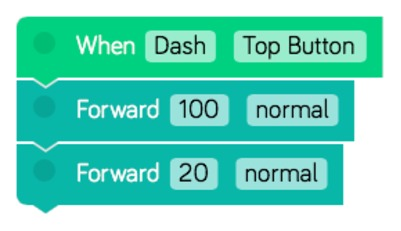### ·         Line up Dash at 0, making sure the back wheel is straight.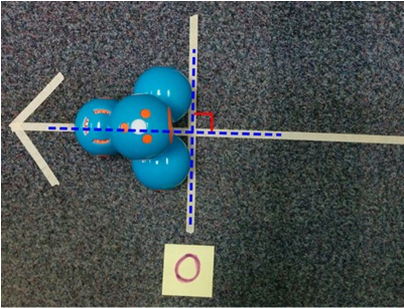·         Select “normal” speed for Dash in your algorithms. This ensures that Dash will travel the required length.

·         Press Dash’s top button. Mark a tick mark at the location Dash stops (create a line of tape in front of Dash as shown in the picture). Do this two more times.

·         Use sticky notes to label the tick marks “0”, “1”, “2”, and “3” (from left to right).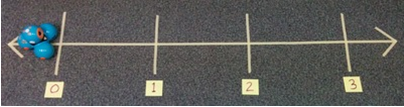·

·         It is important that you use Dash to create the number line, rather than measure 360 cm with a yard stick. This is because Dash may not travel exactly 360 cm, but the other measurements at the same speed will be proportional.

·         Before students enter the class, label the number line with a few fractions.

o   Write Blockly code to make Dash move 40 cm (⅓ unit) and then make a sound when you press Dot’s top button. Line up Dash at 0, making sure the back wheel is straight. Press Dot’s top button 5 times, waiting for Dash to finish moving before each button press. Place a tick mark at Dash’s final location and label it “5/3”.

o   Write Blockly code to make Dash move 30 cm (¼ unit) and then make a sound when you press Dot’s top button. Reset Dash and press Dot’s top button 7 times, waiting for Dash to finish moving before each button press. Place a tick mark at Dash’s final location and label it “7/4”.

o   Write Blockly code to make Dash move 20 cm (⅙ unit) and then make a sound when you press Dot’s top button. Reset Dash and press Dot’s top button 11 times, waiting for Dash to finish moving before each button press. Place a tick mark at Dash’s final location and label it “11/6”.

·         When class begins, write the fraction 5/3 on the board. Ask students “How much does this fraction represent?”

o   The fraction represents “five-thirds” or “one and two-thirds”.

·

### 1.4.2Second Activity: Applaud for Dash Part 2 (25 - 30 minutes)

Before class begins, use masking tape to build a 360-cm-long number line on the floor at the front of the class.

·         See Activity 1 for details on how to build this number line (or use number line from activity).

·         Add minor tick marks at thirds.

·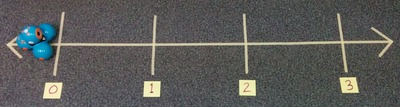·         Write Blockly code to make Dash move 80 cm (⅔ units) and make a sound each time Dot’s top button is pressed.

·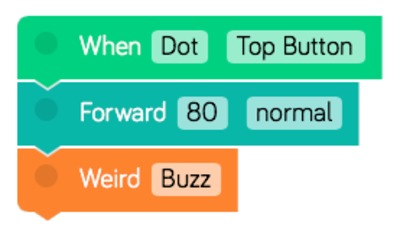·         When class begins, write the equation “5 x (2/3) = ?” on the board. Ask students “How would you model this equation using Dash to find the solution?”

Students should suggest moving Dash 2/3 unit with each button press, and then press the button 5 times.

If students aren’t sure, remind them of the “When Dot Says Go Part 1” activity where they multiplied a whole number, n, by a fraction by pressing Dot’s top button n times.

·         Line up Dash at 0, making sure the back wheel is straight. Remind the students that Dash will move 2/3 unit and make a sound each time Dot’s top button is pressed.

·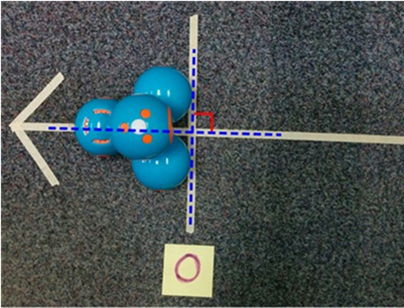·         Ask the class to count the number of button presses.

Use the Blockly code to make Dash move 80 cm (2/3 unit) and make a sound each time Dot’s top button is pressed.

Press Dot’s top button 5 times. Dash should stop at 10/3 on the number line. Label this tick mark “10/3”.

Write 5 x ⅔ = 10/3 on the board.

·         Make sure that Dash has finished moving before pressing the button again.

·         Write “? x ⅓ = 10/3” on the board. Ask the students “How many times will Dash move to get to 10/3 if Dash moves 1/3 unit for each button press?”

Dash will move 10 times.

Students should already know the answer, but if they need to be convinced, program a second Dash to move 40 cm (⅓ unit) and make a sound each time the top button is pressed and demonstrate for the class.

When students answer correctly, write “10 x ⅓ = 10/3” on the board.

·         Ask students to compare the equations “5 x ⅔ = 10/3” and “10 x ⅓ = 10/3”. What do they notice?

Students should notice that both multiplication problems are equal to 10/3.

Students may notice that 5 x 2 = 10 and 10 x 1 = 10. If they don’t notice this yet, do not give it away. There will be more examples where they may start to see a pattern.

·         Now write the equation “3 x (3/4) = ?” on the board. Ask students “How would you model this equation using Dash to find the solution?”

Students should suggest to make Dash move 3/4 unit with each button press, and then press the button 3 times.

·         Remove the minor tick marks from the number line and place new minor tick marks at fourths.

·         Reset Dash and remind the students that Dash will move 3/4 units and make a sound each time Dot’s top button is pressed.

Use the Blockly code to make Dash move 90 cm (3/4 unit) and make a sound each time Dot’s top button is pressed.

Press Dot’s top button 3 times. Dash should stop at 9/4 on the number line. Label this tick mark “9/4”.

Write “3 x (3/4) = 9/4” on the board.

·         Write “? x 1/4 = 9/4” on the board. Ask the students “How many times will Dash move to get to 9/4 if Dash moves 1/4 unit for each button press?”

Dash will move 9 times.

Students should already know the answer, but the teacher can program a second Dash to move 30 cm (¼ unit) with each button press to demonstrate for the class.

When students answer correctly, write “9 x 1/4 = 9/4” on the board.

·         Ask students to compare the equations “3 x ¾ = 9/4” and “9 x ¼ = 9/4”. What do they notice?

Students should notice that both multiplication problems are equal to 9/4.

Students may notice that 3 x 3 = 9 and 9 x 1 = 9. If they don’t notice this yet, do not give it away. There will be another example where they may start to see a pattern.

·         Now write the equation “3 x (5/6) = ?” on the board. Ask students “How would you model this equation using Dash to find the solution?”

Students should suggest to make Dash move 5/6 unit with each button press, and then press the button 3 times.

·         Remove the minor tick marks from the number line and place new minor tick marks at sixths.

·         Reset Dash and remind the students that Dash will move 5/6 units and make a sound each time Dot’s top button is pressed.

Use the Blockly code to make Dash move 100 cm (5/6 unit) and make a sound each time Dot’s top button is pressed.

Press the button 3 times. Dash should stop at 15/6 on the number line. Label this tick mark “15/6”.

Write “3 x (5/6) = 15/6” on the board.

·         Write “? x 1/6 = 15/6” on the board. Ask the students “How many times will Dash move to get to 15/6 if Dash moves 1/6 unit for each button press?”

Dash will move 15 times.

Students should already know the answer, but the teacher can program a second

Dash to move 20 cm (⅙ unit) with each button press and demonstrate for the class.

When students answer correctly, write “15 x 1/6 = 15/6” on the board.

·         Ask students to compare the equations “3 x (5/6) = 15/6” and “15 x 1/6 = 15/6”. What do they notice?

Students should notice that both multiplication problems are equal to 15/6.

Students should notice that 3 x 5 = 15 and 15 x 1 = 15.

·         Tell students to look at the equations on the board. Ask students to explain any patterns that they see.

The pattern is A x (B/C) = (A x B) x (1/C) = (A x B)/C.

This means that to multiply a fraction by a whole number, multiply the numerator of the fraction by the whole number and put this product over the denominator.

If students are struggling to see a pattern, ask them to list all factors of 10, 9, and 15 and then look for a pattern in the equations.

·         Finish the class by confirming that when multiplying a fraction by a whole number, you must multiply the numerator of the fraction by the whole number and put this product over the denominator.

### 1.4.3Third Activity: A Fraction of a Story (20 - 30 minutes)

·         Before class begins, use masking tape to build a 360-cm-long number line on the floor at the front of the class. (or use number line from prior activity)

·         See Activity 1 for details on how to build the number line.·         Divide the class into groups of 2 – 4. Give each group 1 Dash, 1 Blockly compatible tablet, 1 Word Problem Information Sheet and 1 Writing a Word Problem Sheet.

·         Instruct each group to write a word problem, starring Dash, that involves multiplying a whole number by a fraction. Tell students that the solution to the word problem must be able to be acted out by Dash.

o   An example word problem could be “Dash lives 4 blocks from school. Each block is ⅔ of a mile long. How many miles from school does Dash live?”

·         The word problems must be able to be acted out on a number line, or in some other creative way.

o   Students could use the number line and make Dash move ⅔ units four times to model the equation 4 x ⅔ = 10/3.

o   Students could use counters that represent ⅓ and use the bulldozer attachment to collect 4 groups of 2 counters to model the equation 4 x ⅔ = 10/3.

·         The Word Problem Information Sheet contains useful information for how measurements on the number line correspond to unit fractions, and tips for students struggling to build the correct Blockly code.

·         When students have completed the Writing a Word Problem Sheet, hand out 1 Solving a Word Problem Sheet to each group. Instruct students to copy their word problem onto the new worksheet and swap sheets with another group.

·         Instruct each group to solve the new word problem, and program Dash to act out the problem and show the solution.

·         Inform students that they will be presenting their results in the next class.

### 1.4.4Fourth Activity: Presentation Time (20 - 30 minutes)

·         Before class begins, use masking tape to build a 360-cm-long number line on the floor at the front of the class. See Activity 1 for details on how to build the number line.

·         Divide the class into the groups from “Class 3: A Fraction of a Story.” Give each group 1 Dash robot and 1 Blockly compatible tablet.

·         Instruct each group to read the word problem out loud and write the equation that represents the problem on the board. Then use Dash to act out the word problem and show the solution.

## 1.5Educational STANDARDS

Common Core Mathematics:

4.NF.4: Apply and extend previous understandings of multiplication to multiply a fraction by a whole number.

4.NF.4a. Understand a fraction a/b as a multiple of 1/b. For example, use a visual fraction model to represent 5/4 as the product 5 × (1/4), recording the conclusion by the equation 5/4 = 5 × (1/4).

4.NF.4b. Understand a multiple of a/b as a multiple of 1/b, and use this understanding to multiply a fraction by a whole number. For example, use a visual fraction model to express 3 × (2/5) as 6 × (1/5), recognizing this product as 6/5. (In general, n × (a/b) = (n × a)/b.)

4.NF.4c. Solve word problems involving multiplication of a fraction by a whole number, e.g., by using visual fraction models and equations to represent the problem. For example, if each person at a party will eat 3/8 of a pound of roast beef, and there will be 5 people at the party, how many pounds of roast beef will be needed? Between what two whole numbers does your answer lie?

Common Core ELA:

W.4.3: Write narratives to develop real or imagined experiences or events using effective technique, descriptive details, and clear event sequences.

W.4.4: Produce clear and coherent writing in which the development and organization are appropriate to task, purpose, and audience.

SL.4.4: Report on a topic or text, tell a story, or recount an experience in an organized manner, using appropriate facts and relevant, descriptive details to support main ideas or themes; speak clearly at an understandable pace.

SL.4.1: Engage effectively in a range of collaborative discussions (one-on-one, in groups, and teacher-led) with diverse partners on grade 4 topics and texts, building on others' ideas and expressing their own clearly.